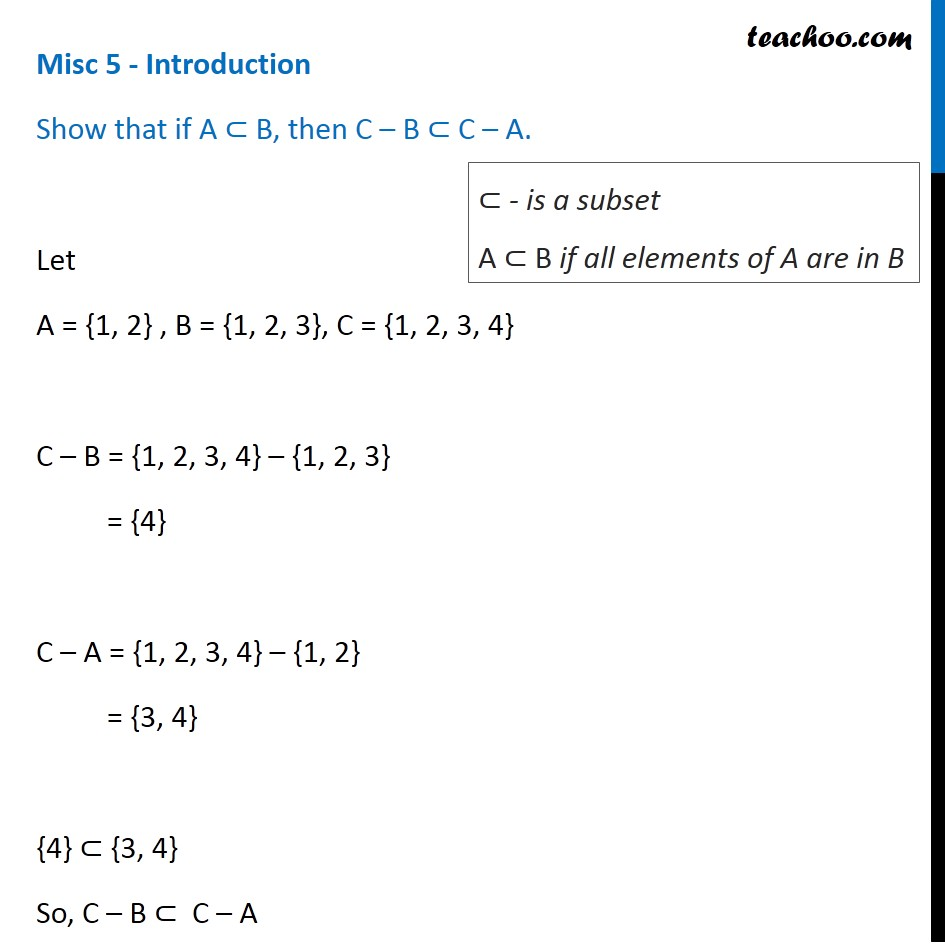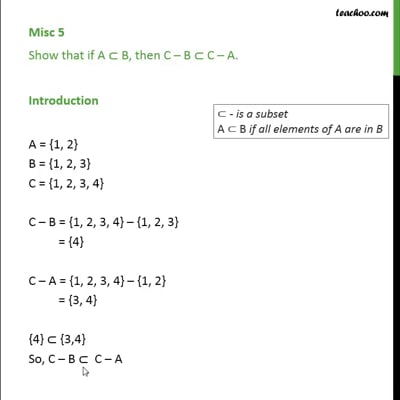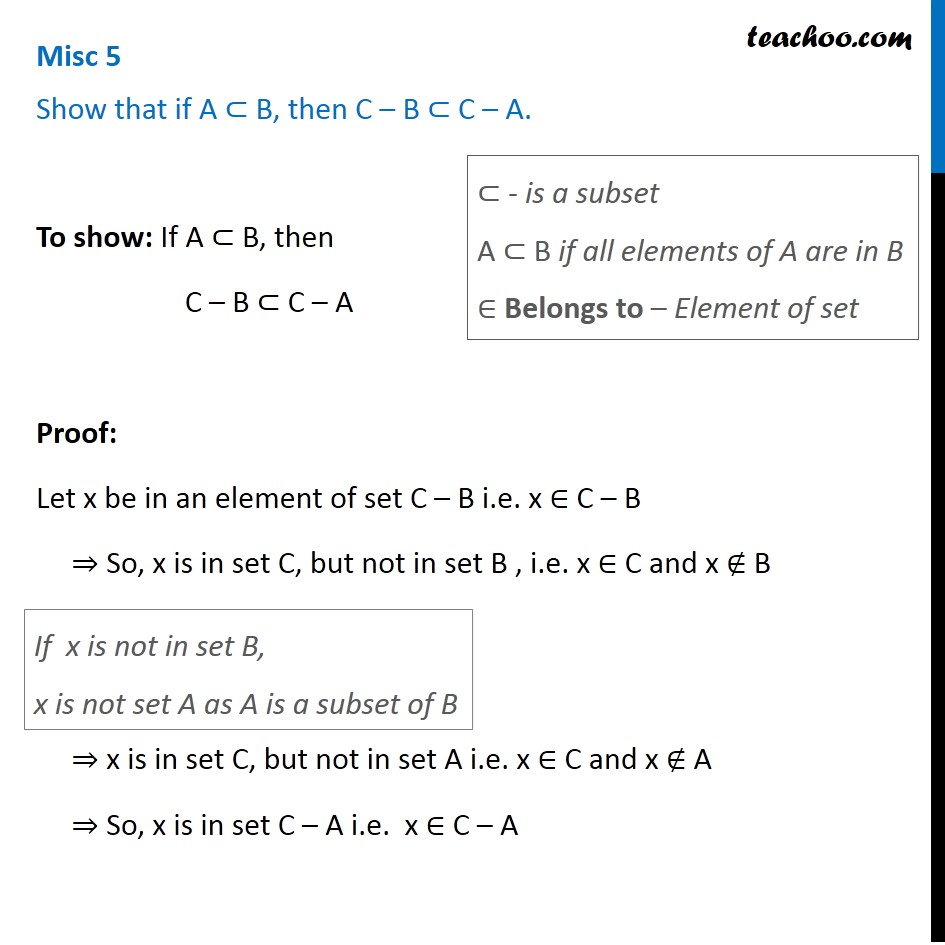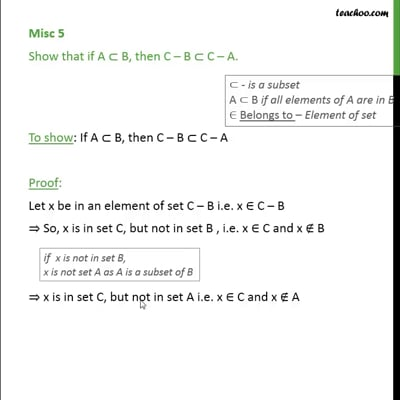Proof - Using properties of sets

Chapter 1 Class 11 Sets
Concept wiseThis video is only available for Teachoo black usersThis video is only available for Teachoo black users

Introducing your new favourite teacher - Teachoo Black, at only ₹83 per month

### Transcript

Misc 5 - Introduction Show that if A ⊂ B, then C – B ⊂ C – A. Let A = {1, 2} , B = {1, 2, 3}, C = {1, 2, 3, 4} C – B = {1, 2, 3, 4} – {1, 2, 3} = {4} C – A = {1, 2, 3, 4} – {1, 2} = {3, 4} {4} ⊂ {3, 4} So, C – B ⊂ C – A ⊂ - is a subset A ⊂ B if all elements of A are in B Misc 5 Show that if A ⊂ B, then C – B ⊂ C – A. To show: If A ⊂ B, then C – B ⊂ C – A Proof: Let x be in an element of set C – B i.e. x ∈ C – B ⇒ So, x is in set C, but not in set B , i.e. x ∈ C and x ∉ B ⇒ x is in set C, but not in set A i.e. x ∈ C and x ∉ A ⇒ So, x is in set C – A i.e. x ∈ C – A If x is not in set B, x is not set A as A is a subset of B ⊂ - is a subset A ⊂ B if all elements of A are in B ∈ Belongs to – Element of set ∴ If x ∈ C – B ,then x ∈ C – A i.e. If an element belongs to the set C – B , it also belongs to the set C – A ⇒ C – B ⊂ C – A Hence proved